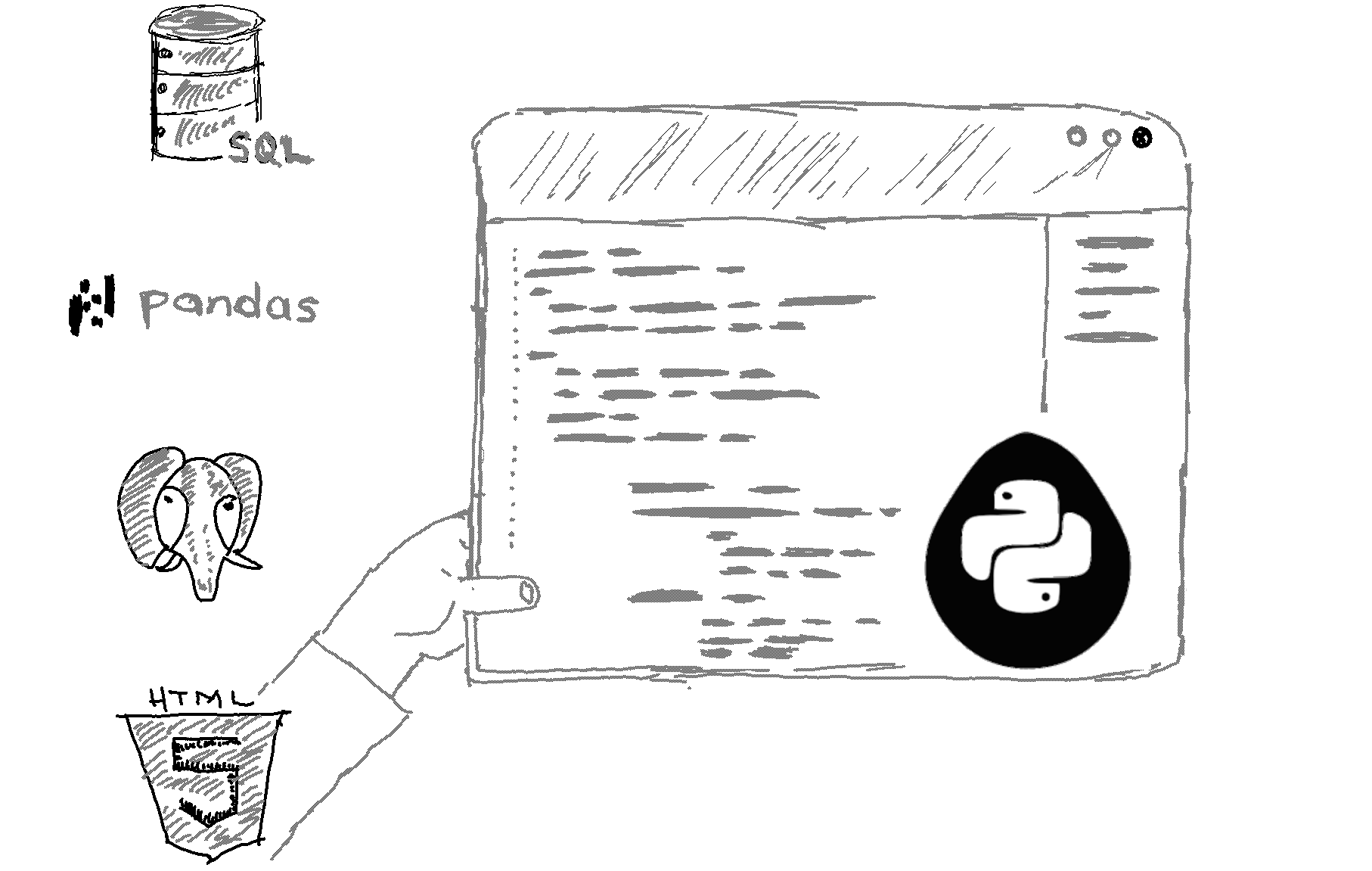# Here is how to create a dictionary with list comprehension in Python.

To create a dictionary using list comprehension in Python, you can follow these steps:

1. Define your list or iterable from which you want to create the dictionary.

2. Use list comprehension to generate key-value pairs in the form of tuples.

3. Convert the list of tuples into a dictionary using the dict() constructor.

Here's an example that demonstrates this process:

```# Example 1: Creating a dictionary from a list of values
my_list = ['apple', 'banana', 'cherry', 'date']
my_dict = {value: len(value) for value in my_list}
print(my_dict) ```

Output

{'apple': 5, 'banana': 6, 'cherry': 6, 'date': 4}

Explanation

In this example, we created a dictionary where the keys are the elements from the my_list and the values are the lengths of the corresponding elements.

You can also create dictionaries from two separate lists using list comprehension. Here's an example:

```# Example 2: Creating a dictionary from two lists
keys = ['name', 'age', 'city']
values = ['John', 25, 'New York']

my_dict = {keys[i]: values[i] for i in range(len(keys))}
print(my_dict)
```
This outputs:
`{'name': 'John', 'age': 25, 'city': 'New York'}`
In this example, we paired the elements at corresponding indices from the keys and values lists to create a dictionary.

Using list comprehension in this way provides a concise and efficient method to create dictionaries from iterable objects in Python.

Related HowTos
##### Recommended Course

Learn Flask development and learn to build cool apps with our premium Python course on Udemy.